# Functions whose Inverses are Functions

Recall that we defined inverses for any relation:

Definition. If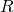is a relation from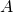to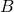, then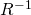is a relation fromto, defined by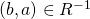iff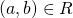.

Thus (in our formalism) every function has an inverse. The inverse of a function so defined, however, may not be a function itself! (Remember that most operations we do to sets or to relations will not preserve functionhood.) So let's discover when taking the inverse (swapping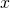and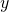") actually does result in a function.

Recall that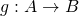means that two conditions hold:

1.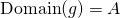2.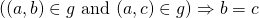.

Exercise 1. Suppose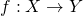. By substituting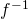for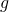,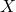for, and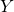for, write what it means for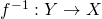.

Exercise 2. Use the definition ofto rewrite your conditions solely in terms of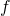(with noanywhere).

Definition.  Let. We callonto or surjective if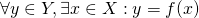. We callone-to-one or injective if for any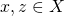, we have that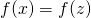guarantees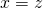.

Definition. A function which is both one-to-one and onto is called a one-to-one correspondence or a bijection.

The following theorem is important, but its proof is just the combination of Exercises 1 and 2.

Theorem. Let. Theniffis both one-to-one and onto.

Theorem.  Supposeis one-to-one and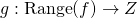is one-to-one. Then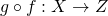is one-to-one.

Proof. Note that the condition thatbe defined on the range ofis necessary in order to compose the two functions as functions.

Let. Suppose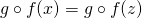. Applying the fact that the composition of functions is a function, we haveSinceis one-to-one, we have. Sinceis one-to-one, we have. Since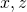were arbitrary, we've shown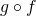is one-to-one.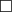.

Theorem. Supposeis onto and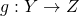is onto. Thenis onto.

Proof. This is an exercise for you.